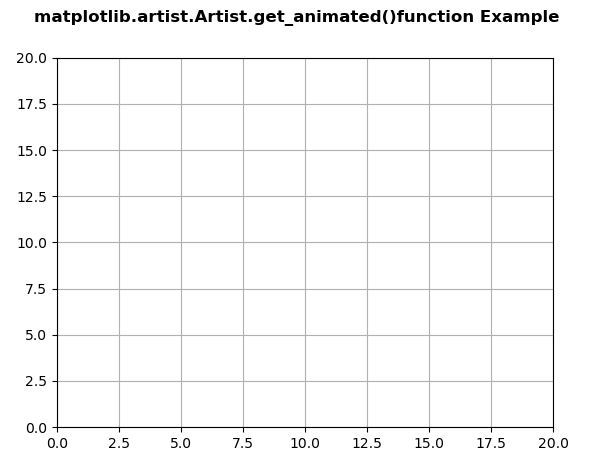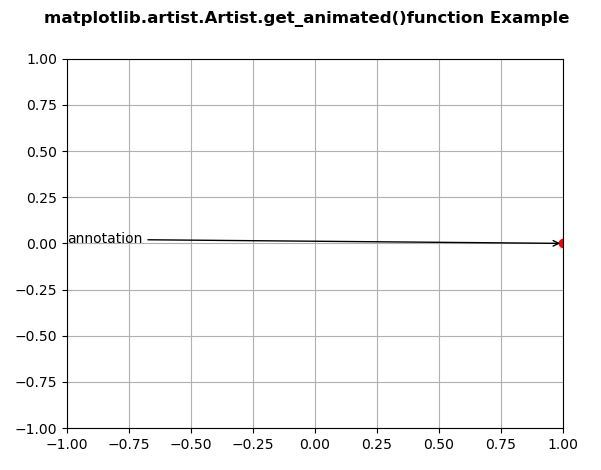Related Articles

# Matplotlib.artist.Artist.get_animated() in Python

• Last Updated : 10 May, 2020

Matplotlib is a library in Python and it is numerical – mathematical extension for NumPy library. The Artist class contains Abstract base class for objects that render into a FigureCanvas. All visible elements in a figure are subclasses of Artist.

## matplotlib.artist.Artist.get_animated() method

The get_animated() method in artist module of matplotlib library is used to get the animated state.

Syntax: Artist.get_animated(self)

Parameters: This method does not accepts any parameter.

Returns: This method return the the animated state.

Below examples illustrate the matplotlib.artist.Artist.get_animated() function in matplotlib:

Example 1:

 `# Implementation of matplotlib function``from` `matplotlib.artist ``import` `Artist  ``import` `numpy as np ``import` `matplotlib.pyplot as plt ``import` `matplotlib.animation as animation ``   ` `    ` `data ``=` `np.array([[``1``, ``2``, ``3``, ``4``, ``5``],  ``                ``[``7``, ``4``, ``9``, ``2``, ``3``]]) ``    ` `fig ``=` `plt.figure() ``ax ``=` `plt.axes(xlim ``=``(``0``, ``20``), ylim ``=``(``0``, ``20``)) ``    ` `line, ``=` `ax.plot([], [], ``'r-'``) ``annotation ``=` `ax.annotate('', xy ``=``(data[``0``][``0``],  ``                         ``data[``1``][``0``])) `` ` `Artist.set_animated(annotation, ``True``) ``w ``=` `Artist.get_animated(annotation) ``   ` `print``(``str``(w)) `` ` `fig.suptitle('matplotlib.artist.Artist.get_animated()\``function Example', fontweight ``=``"bold"``) `` ` `ax.grid()`` ` `plt.show()`

Output:`True`

Example 2:

 `# Implementation of matplotlib function``from` `matplotlib.artist ``import` `Artist  ``import` `numpy as np ``import` `matplotlib.pyplot as plt ``import` `matplotlib.animation as animation ``    ` `   ` `fig, ax ``=` `plt.subplots() ``    ` `ax.set_xlim([``-``1``, ``1``]) ``ax.set_ylim([``-``1``, ``1``]) ``    ` `L ``=` `50``theta ``=` `np.linspace(``0``, ``2` `*` `np.pi, L) ``r ``=` `np.ones_like(theta) ``    ` `x ``=` `r ``*` `np.cos(theta) ``y ``=` `r ``*` `np.sin(theta) ``    ` `line, ``=` `ax.plot(``1``, ``0``, ``'ro'``) ``    ` `annotation ``=` `ax.annotate( ``    ``'annotation'``, xy ``=``(``1``, ``0``), xytext ``=``(``-``1``, ``0``), ``    ``arrowprops ``=` `{``'arrowstyle'``: ``"->"``} ``) ``Artist.set_animated(annotation, ``False``) ``w ``=` `Artist.get_animated(annotation) ``   ` `print``(``str``(w)) `` ` `fig.suptitle('matplotlib.artist.Artist.get_animated()\``function Example', fontweight ``=``"bold"``) `` ` `ax.grid()`` ` `plt.show()`

Output:`False`

Attention geek! Strengthen your foundations with the Python Programming Foundation Course and learn the basics.

To begin with, your interview preparations Enhance your Data Structures concepts with the Python DS Course. And to begin with your Machine Learning Journey, join the Machine Learning – Basic Level Course

My Personal Notes arrow_drop_up